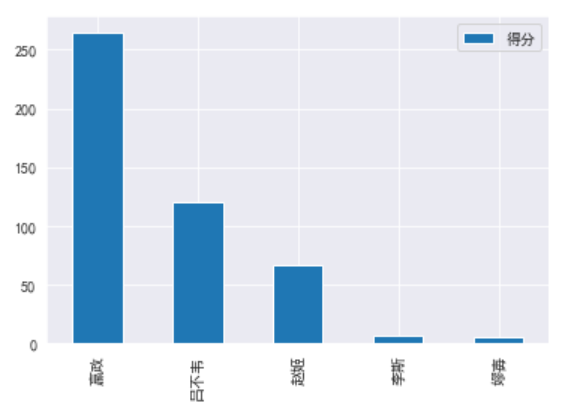汽车站旁

0571-98765432

1、 数据抓取

``scrapy startproject daqinfu``scrapy genspider daqinfu "douban.com"``

，其中start是获取评论的起始位置，limit代表获取多少条评论数据。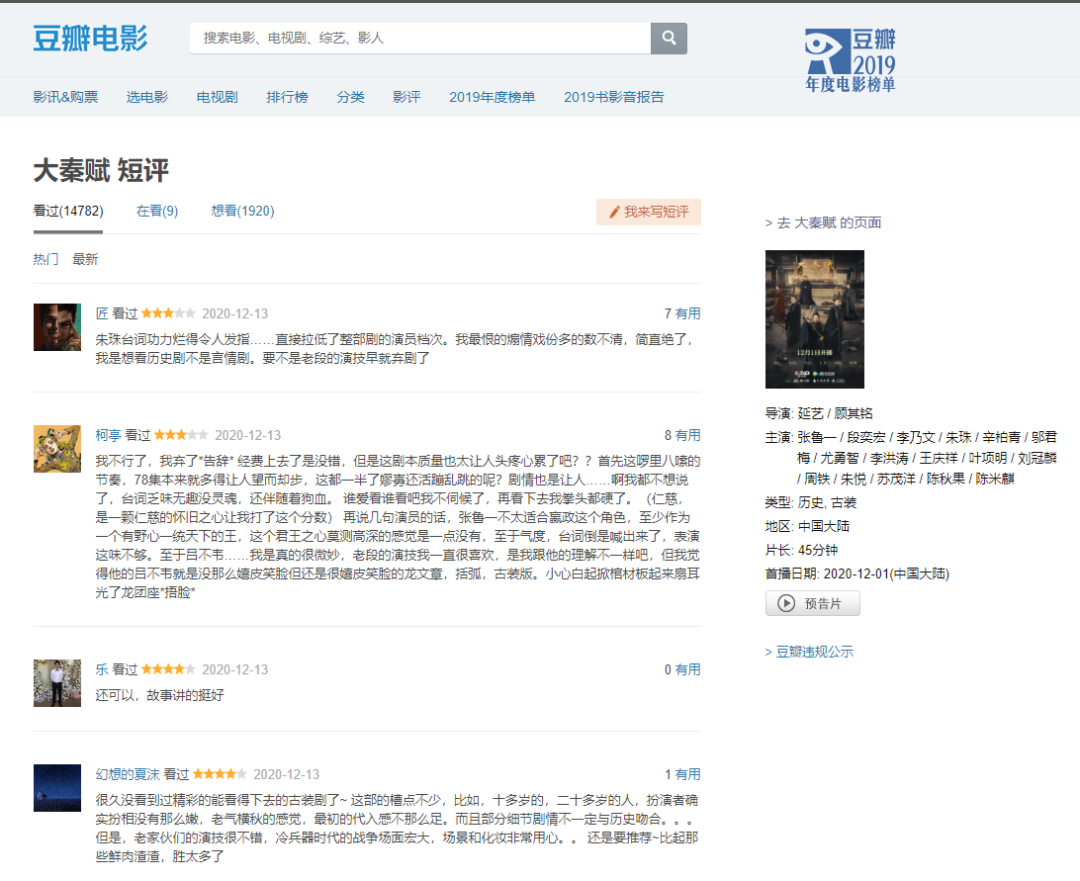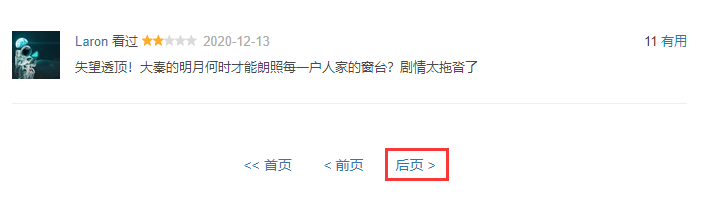``# -*- coding: utf-8 -*-``import re``from ..items import QinItem``class QinSpider(scrapy.Spider):`` name = 'qin'`` allowed_domains = ['douban.com']`` start_urls = ['https://movie.douban.com/subject/26413293/comments?start=0&limit=20']`` domain = "https://movie.douban.com/subject/26413293/comments"`` def parse(self, response):`` comment_items = response.xpath("//div[@id='comments']/div[contains(@class,'comment-item')]")`` for comment_item in comment_items:`` pub_time = comment_item.xpath(".//span[contains(@class,'comment-time')]/@title").extract_first`` rating_classes = comment_item.xpath(".//span[contains(@class,'rating')]/@class").extract_first`` rating = re.search(r'allstar(\d)0 rating',rating_classes).group(1)`` content = comment_item.xpath(".//p[contains(@class,'comment-content')]/span/text").extract_first`` item = QinItem(pub_time=pub_time,rating=rating,content=content)`` yield item`` query = response.xpath("//div[@id='paginator']/a[@class='next']/@href").extract_first`` if query:`` yield scrapy.Request(url=self.domain+query,callback=self.parse)`` print("="*40)`` print(response.url,query)`` print("=" * 40)``

``import json`` def __init__(self):`` self.fp = open("comments.json", 'w', encoding='utf-8')`` def process_item(self, item, spider):`` self.fp.write(json.dumps(dict(item))+"\n")`` return item`` def close_spider(self,spider):`` self.fp.close``

2、数据分析：

``items = ``with open("comments.json","r") as fp:`` for line in fp:`` comment = json.loads(line)`` items.append(comment)``
``item_list = [[item['pub_time'],item['rating'],item['content']] for item in items]``comment_df = pd.DataFrame(item_list,columns=['pub_time','rating','content'])``comment_df['pub_time'] = pd.to_datetime(comment_df['pub_time'])``comment_df.head``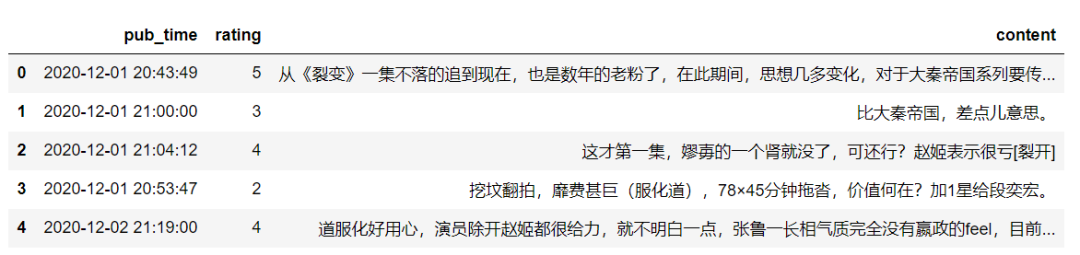2.1. 时间分析：

``# 分析评论日期``from matplotlib import dates``
``plt.figure(figsize=(10,5))``
``# 2. 添加一个新的pub_date``comment_df['pub_date'] = comment_df['pub_time'].dt.date``# # 3. 根据日期分组绘图``comment_date_df = comment_df.groupby(['pub_date']).count``ax = sns.lineplot(x=comment_date_df.index,y=comment_date_df.content,marker='o')``# 设置显示所有时间``ax.set(xticks=comment_date_df.index)``# 设置x轴旋转``_ = ax.set_xticklabels(comment_date_df.index,rotation=45)``# 设置x轴格式``ax.xaxis.set_major_formatter(dates.DateFormatter("%m-%d"))``ax.set_xlabel("发布日期")``ax.set_ylabel("评论数量")``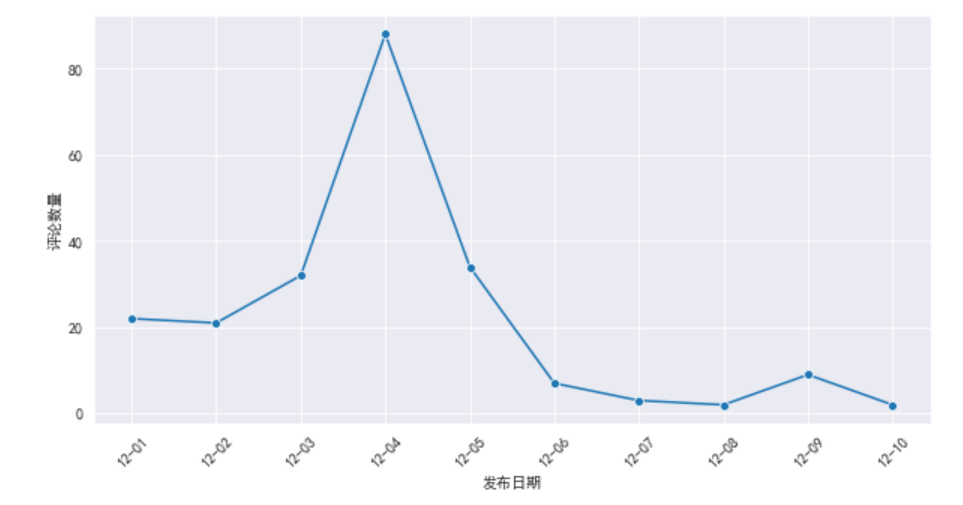``# 分析评论时间``time_range = [0,2,4,6,8,10,12,14,16,18,20,22,24]``comment_time_df = comment_df['pub_time'].dt.hour``time_range_counts = pd.cut(comment_time_df,bins=time_range,include_lowest=True,right=False).value_counts``ax = time_range_counts.plot(kind="bar")``_ = ax.set_xticklabels(labels=time_range_counts.index,rotation=45)``ax.set_xlabel("发布时间")``ax.set_ylabel("发布数量")``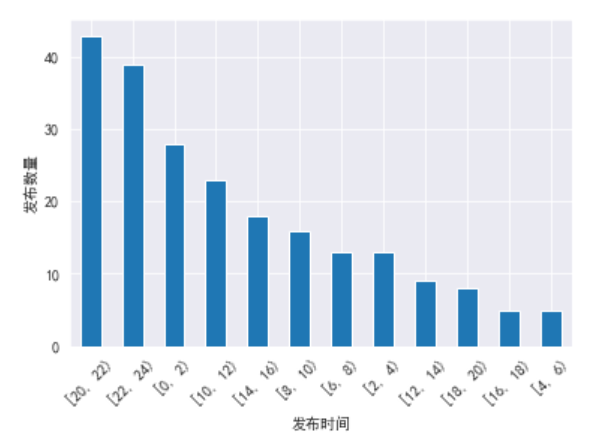2.2. 评分分析：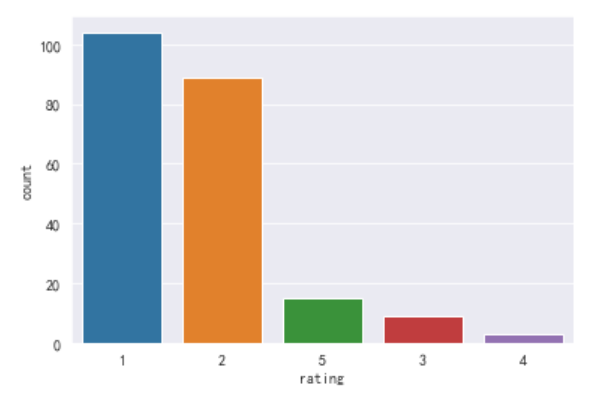# 评论数据

2.3. 分析人物评分

``# 电视剧人物的评分``import jieba``roles = {'嬴政':0,'秦始皇':0,'张鲁一':0,'吕不韦':0,'段奕宏':0,'李斯':0,'嫪毐':0,"赵姬":0,"朱珠":0}``role_names = list(roles.keys)``for name in role_names:`` jieba.add_word(name)``for row in comment_df.index:`` rating = comment_df.loc[row,'rating']`` if rating:`` content = comment_df.loc[row,"content"]`` words = list(jieba.cut(content, cut_all=False))`` names = set(role_names).intersection(set(words))`` for name in names:`` roles[name] += int(rating)``roles['嬴政'] += roles['嬴政'] + roles['秦始皇'] + roles['张鲁一']``roles['吕不韦'] += roles['吕不韦'] + roles['段奕宏']``roles['李斯'] += roles['李斯']``roles['赵姬'] += roles['赵姬'] + roles['朱珠']``
``roles.pop("秦始皇")``roles.pop("张鲁一")``roles.pop("段奕宏")``roles.pop("朱珠")``
``role_df = pd.DataFrame(list(roles.values),index=list(roles.keys),columns=['得分'])``role_df.sort_values('得分',inplace=True,ascending=False)``role_df.plot(kind='bar')``